# Pytorch学习笔记1

## 对Pytorch的初步了解

### 简介

PyTorch是一个开源的Python机器学习库，基于Torch，用于自然语言处理等应用程序。
1、具有强大的GPU加速的张量计算（如NumPy）。
2、包含自动求导系统的的深度神经网络。

### 发展

PyTorch的前身是Torch，其底层和Torch框架一样，但是使用Python重新写了很多内容，不仅更加灵活，支持动态图，而且提供了Python接口。它是由Torch7团队开发，是一个以Python优先的深度学习框架，不仅能够实现强大的GPU加速，同时还支持动态神经网络，这是很多主流深度学习框架比如Tensorflow等都不支持的。

### 优点

PyTorch是相当简洁且高效快速的框架

PyTorch作者亲自维护的论坛 供用户交流和求教问题

# 构造一个未初始化的5X3的矩阵


x=torch.empty(5,3)
x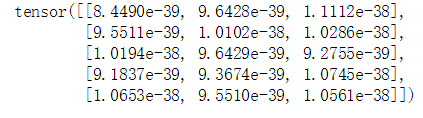# 构建一个初始化的矩阵(随机数一般在0,1之间)


x=torch.rand(5,3)
x

tensor([[0.0412, 0.9912, 0.7759],
[0.4840, 0.2176, 0.1873],
[0.9202, 0.3406, 0.9724],
[0.9870, 0.6207, 0.4252],
[0.4820, 0.2094, 0.6577]])


# 构建一个全部为0的类型为long的矩阵


x=torch.zeros(5,3,dtype=torch.long)

print(x.dtype)
x

torch.int64
tensor([[0, 0, 0],
[0, 0, 0],
[0, 0, 0],
[0, 0, 0],
[0, 0, 0]])



# 数据类型转化

x=torch.zeros(5,4).long()#强制转化
x.dtype

torch.int64


# 从数据里面直接构建tensor，查看数据类型


x=torch.tensor([5.5,3])
x.dtype

torch.float32


# 也可从一个已经存在的tensor构建一个rensor，这些方法会重用原来的tensor的特征，例如，数据类型，除非提供新的数据

x=x.new_ones(5,3)
x.dtype#可以发现这里数据类型和上面的X是一样的都是float32

torch.float32


# 如果我们需要改变数据类型


x=x.new_ones(5,3,dtype=torch.int)
x

tensor([[1, 1, 1],
[1, 1, 1],
[1, 1, 1],
[1, 1, 1],
[1, 1, 1]], dtype=torch.int32)


# 随机产生一个和x形状相同的tensor

x=torch.randn_like(x,dtype=torch.float)
x

tensor([[ 0.5025,  0.1676, -1.1412],
[-0.9381,  0.8245, -0.2424],
[-2.7374, -1.8640,  0.1465],
[ 0.4347,  0.2672,  1.0185],
[-0.0883, -0.8289, -0.3539]])


# 注意troch.size返回的是一个tuple

#如果想知道tensor的形状我们可以
x.size()
#注意troch.size返回的是一个tuple

torch.Size([5, 3])


# 注意任何in-place的运算符会以_结尾，举例来说


x.copy_(y)
x

tensor([[ 1.3537,  0.4845, -0.3104],
[-0.8712,  1.8012, -0.0918],
[-2.7261, -1.8565,  0.9825],
[ 1.1298,  0.7133,  1.5895],
[ 0.7637, -0.5226, -0.2892]])


# 各种类似Numpy的indexing都可以在PyTorch tensor上面使用


x[0:3,0:5]

tensor([[ 1.3537,  0.4845, -0.3104],
[-0.8712,  1.8012, -0.0918],
[-2.7261, -1.8565,  0.9825]])


# 如果你希望resize一个tensor，可以使用torch.view,在numpy里面是reshape


x=torch.rand(4,4)
y=x.view(2,8)
y

tensor([[0.4772, 0.2789, 0.6982, 0.4009],
[0.8561, 0.4847, 0.3435, 0.3529],
[0.1766, 0.3924, 0.7261, 0.8916],
[0.1214, 0.6097, 0.0264, 0.4717]])


# 如果你有一个只有一个元素的tensor，使用.item()方法可以把里面的value变成Python数值


x=torch.randn(1)
x.item()

-0.9713826179504395

import numpy as np
a=np.ones(5)#生成五个一
b=torch.from_numpy(a)
a

array([7., 7., 7., 7., 7.])
In :



## 用numpy和pytorch来完成一个简单的没有偏置的小神经网络

### numpy实现

#### 关于里面矩阵求导转置的说明：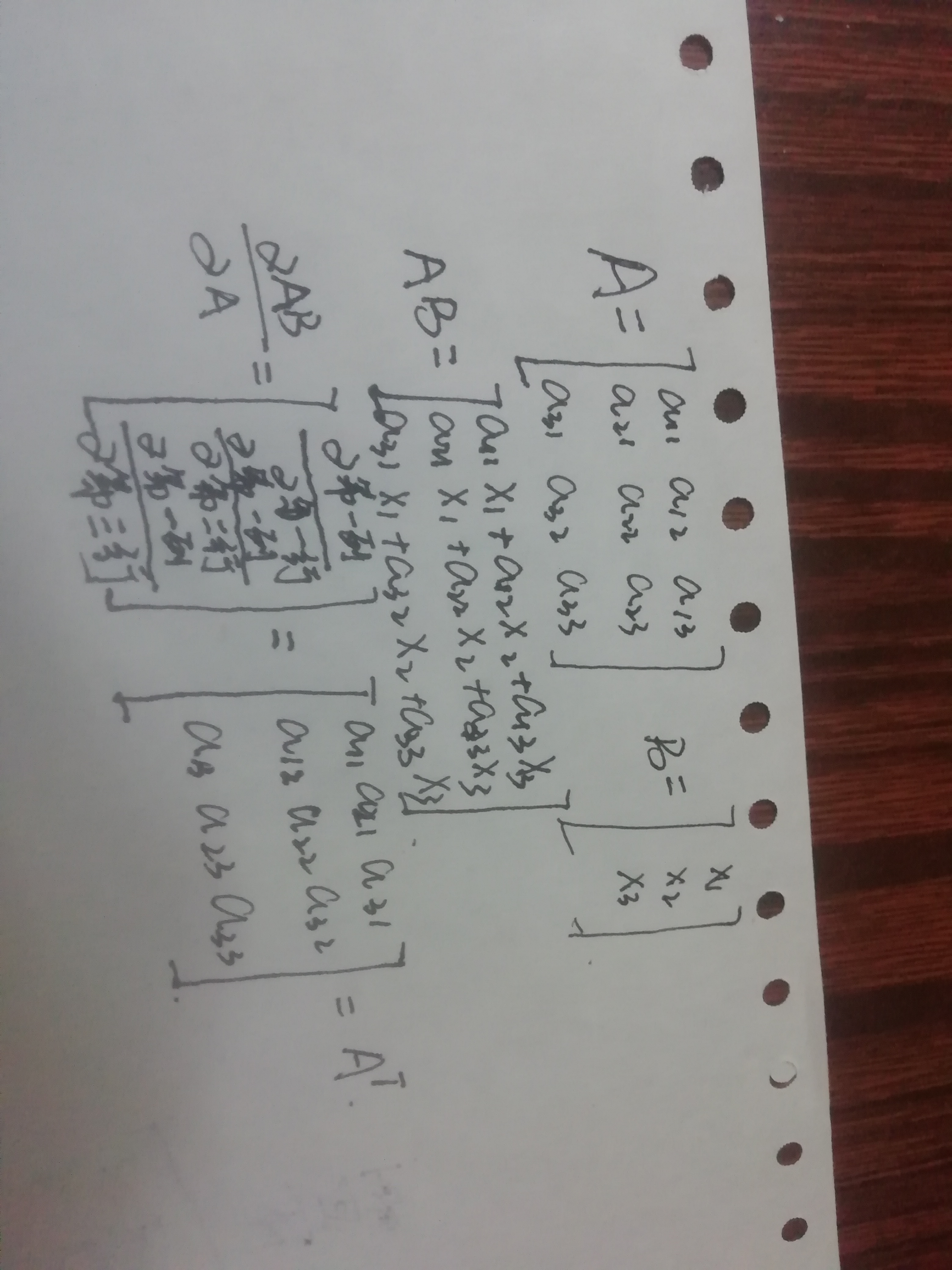#N:多少个样本数据
#D_in：输入的维度
#H : 隐藏层的节点数
#D_out:输出的维度，输出的节点数
import numpy as np

'''
numpy.random.rand()产生从[0,1)之间的随机数，没有负值。
numpy.random.randn()产生服从正态分布的随机数，会出现负值。
'''

N,D_in,H,D_out=64,1000,100,10
#创建随机的输入数据和输出数据
x=np.random.randn(N,D_in)
y=np.random.randn(N,D_out)

#w是个可以把数据从1000维度变成100维度的
#生成一个1000*100的矩阵
w1=np.random.randn(D_in,H)#(1000,100)，输入层到隐藏层的权值
w2=np.random.randn(H,D_out)#(100,10)，隐藏层带输出层的权重

#学习率
learning_rate= 1e-6# 1*10^6
for it in range(500):
#forword pass
#.dot()矩阵乘法
#当前对应隐藏层矩阵的值
h=x.dot(w1)# N*H·
h_relu=np.maximum(h,0)#N*H  取出特征中最大的
y_pred=h_relu.dot(w2)#H *D_out 求得我们的预测值

#compute lose
loss=np.square(y_pred-y).sum()#用目标值-预测值求得均方差
print(it,loss)#输出对应的轮数和损失值

#Backward pass
#反向传播，梯度下降法
#loss=np.square(y_pred-y).sum()对y_pred的求导
#矩阵求导公式  d AB/ d B= A.T
#预测值对w2 隐藏层到输出层的权值
#权值对于卷积的求导
#根据函数图像可以看出再小于零的时候导数是为0的
#求出了w1的导数

#update weights of wl and w2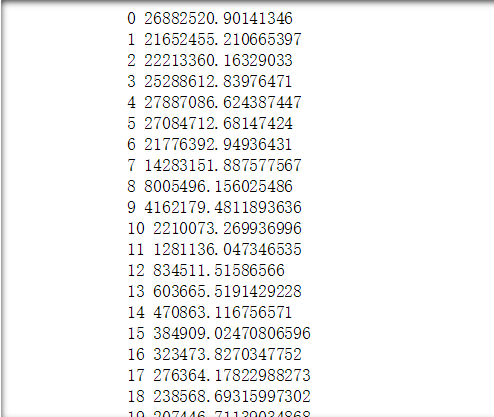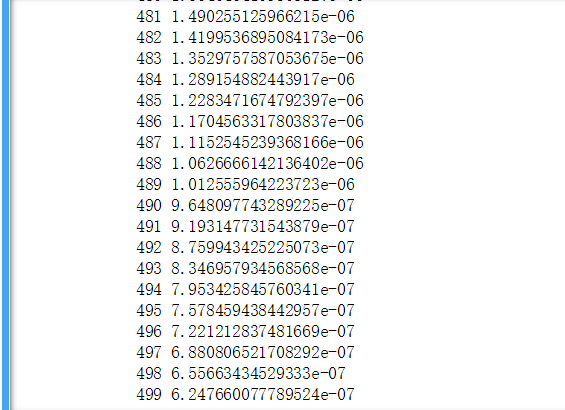### 接下来试一下pytorch实现

numpy和pytorch不一样的地方做一下修改
N:多少个样本数据
D_in：输入的维度
H : 隐藏层的节点数
D_out:输出的维度，输出的节点数
numpy改成torch
numpy.random.randn--->torch.randn
dot-->mm
np.maximum(h,0)-->h.clamp(min=0)
np.square（x-y）-->(x-y).pow(2).item()
x.T-->x.t()
x.copy()---> x.clone()

import torch
N,D_in,H,D_out=64,1000,100,10
#创建随机的输入数据和输出数据
x=torch.randn(N,D_in)
y=torch.randn(N,D_out)

#w是个可以把数据从1000维度变成100维度的
#生成一个1000*100的矩阵
w1=torch.randn(D_in,H)#(1000,100)，输入层到隐藏层的权值
w2=torch.randn(H,D_out)#(100,10)，隐藏层带输出层的权重

#学习率

learning_rate= 1e-6# 1*10^6
for it in range(500):
#forword pass
#.mm()矩阵乘法
#当前对应隐藏层矩阵的值
h=x.mm(w1)# N*H·
h_relu=h.clamp(min=0)#N*H  取出特征中最大的
y_pred=h_relu.mm(w2)#H *D_out 求得我们的预测值

#compute lose
loss=(y_pred-y).pow(2).sum().item()#用目标值-预测值求得均方差
print(it,loss)#输出对应的轮数和损失值

#Backward pass
#反向传播，梯度下降法
#loss=np.square(y_pred-y).sum()对y_pred的求导
#矩阵求导公式  d AB/ d B= A.T
#预测值对w2 隐藏层到输出层的权值
#权值对于卷积的求导
#根据函数图像可以看出再小于零的时候导数是为0的
#求出了w1的导数

#update weights of wl and w2


### 用torch的backward尽可能简单的实现传统的反向传播神经网络

import torch
N,D_in,H,D_out=64,1000,100,10
#创建随机的输入数据和输出数据
x=torch.randn(N,D_in)
y=torch.randn(N,D_out)
#w是个可以把数据从1000维度变成100维度的
#生成一个1000*100的矩阵
#学习率
learning_rate= 1e-6# 1*10^6
for it in range(500):
#这里直接求出y的预测值
y_pred=x.mm(w1).clamp(min=0).mm(w2)
loss=(y_pred-y).pow(2).sum()#用目标值-预测值求得均方差，这是loss是一个tensor，不是一个数，如打印出来要加item()
print(it,loss.item())#输出对应的轮数和损失值
loss.backward()#用这一句代替上面繁琐的手动求导，backwa会求出链式反应里面的所有导数，以tensor的形式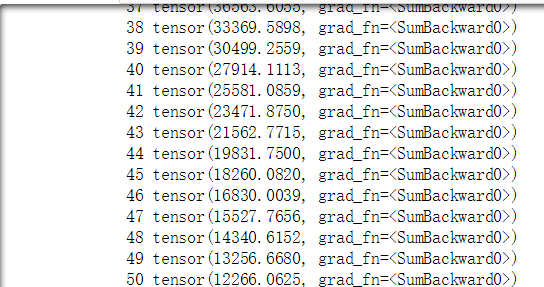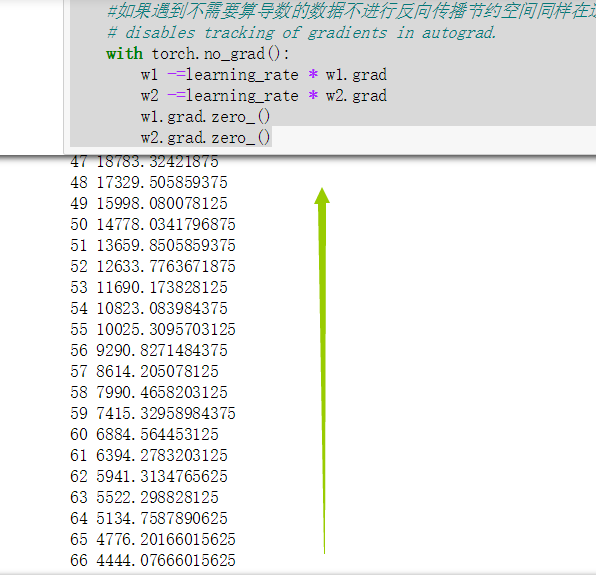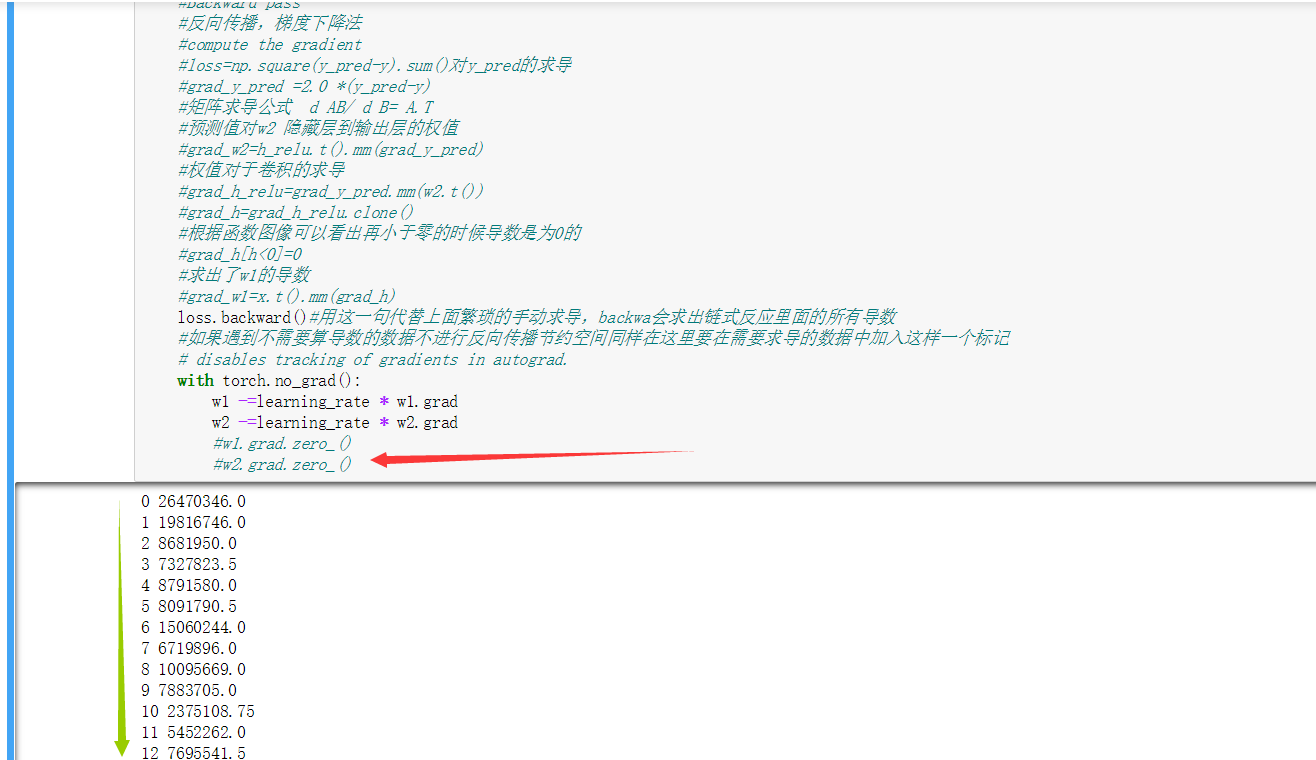posted on 2020-03-03 23:39  那我先走了  阅读(213)  评论(0编辑  收藏  举报